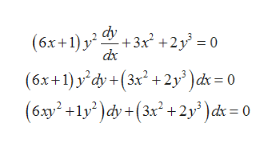# Solve the first order differential equation using any acceptable method.(6x+1)y2 dy/dx+3x2+2y3=0,    y(0)=1

Question
364 views

Solve the first order differential equation using any acceptable method.

(6x+1)y2 dy/dx+3x2+2y3=0,    y(0)=1

check_circle

star
star
star
star
star
1 Rating
Step 1

Given the differential equation is

Now separate the variables as followshelp_outlineImage Transcriptionclosedy (6x+1) y2 3x +2y = 0 dx (6x+1)y'dy+(3x2 +2y?)dk 0 (6ay2 +1y)dy +(3x2 +2y' )ck= 0 fullscreen
Step 2

Then,

Step 3

Then take partial differentiation,

Hence th...

### Want to see the full answer?

See Solution

#### Want to see this answer and more?

Solutions are written by subject experts who are available 24/7. Questions are typically answered within 1 hour.*

See Solution
*Response times may vary by subject and question.
Tagged in

### Math